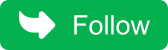## Thursday, May 5, 2022

### Question 99 : Count Factorial Trailing Zeroes in java.

Count number of zeros in factorial of number in java.

For example:
Factorial of 6 is 720, so a number of trailing zeros is 1.
Factorial of 14 is 87 178 291 200, so a number of trailing zeros is 2.

## Solution

A very simple approach is to compute the factorial and divide it by 10 to count a number of trailing zeros but bound of ints will be reached very quickly with solution.

``Trailing zeroes are created by multiple of 10 and multiples of 10 are created by 5 and 2.As multiple of 2 will always more than multiple of 5, we can simply count the multiples of 5.``

Let’s create a simple program to count factorial Trailing Zeroes in java.

``public class FactorialTrailingZeroMain {     public static void main(String[] args)    {        FactorialTrailingZeroMain ftzm=new FactorialTrailingZeroMain();        int countFactorialTrailingZeros = ftzm.countFactorialTrailingZeros(29);         System.out.println("Factorial trailing zeroes for 29: "+countFactorialTrailingZeros);    }     public int countFactorialTrailingZeros(int num)    {        int countOfZeros=0;        for(int i=2;i<=num;i++)        {            countOfZeros+=countFactorsOf5(i);        }        return countOfZeros;    }     private int countFactorsOf5(int i)    {        int count=0;        while(i%5==0)        {            count++;            i/=5;        }        return count;    }}``

When you run above program, you will see below output.

``Factorial trailing zeroes for 29: 6``
This approach works but can we do better?
Yes,we can.

Algorithms to Count number of zeros in factorial of number in java

• Divide the number by 5 and count the number of multiple of 5.
• Divide the number by 25 and count the number of multiple of 25.
• Divide the number by 125 and count the number of multiple of 125.
• Divide the number by 125 and count the number of multiple of 625 and so on …

## Program for Counting Factorial Trailing Zeroes in java

``````public class FactorialTrailingZeroMain {

public static void main(String[] args)
{
FactorialTrailingZeroMain ftzm=new FactorialTrailingZeroMain();
int countFactorialTrailingZeros = ftzm.countFactorialTrailingZeros(29);

System.out.println("Factorial trailing zeroes for 29: "+countFactorialTrailingZeros);
}

public int countFactorialTrailingZeros(int num)
{
int countOfZeros=0;
if(num<0) return -1; for(int i=5;num/i>0;i*=5)
{
countOfZeros+=num/i;
}
return countOfZeros;
}
}
``````
Don't miss the next article!

Be the first to be notified when a new article or Kubernetes experiment is   published.